# Fitting a psychometric curve using pyTorch

The goal here is to compare methods which fit data with psychometric curves using logistic regression. Indeed, after (long) experiments where for instance you collected sequences of keypresses, it is important to infer at best the parameters of the underlying processes: was the observer biased, or more precise?

While I was forevever using sklearn or lmfit (that is, scipy's minimize) and praised these beautifully crafted methods, I sometimes lacked some flexibility in the definition of the model. This notebook was done in collaboration with Jenna Fradin, master student in the lab.

tl; dr = Do not trust the coefficients extracted by a fit without validating for methodological biases.

One part of flexibility I missed is taking care of the lapse rate, that is the frequency with which you just miss the key. In a psychology experiment, you often see a fast sequence of trials for which you have to make a perceptual deccision, for instance press the Left or Right arrows. Sometimes you know the answer you should have done, but press the wrong eror. This error of distraction is always low (in the order of 5% to 10%) but could potentially change the results of the experiments. This is one of the aspects we will evaluate here.

In this notebook, I define a fitting method using pytorch which fits in a few lines of code :

In :
import torch

torch.set_default_tensor_type("torch.DoubleTensor")
criterion = torch.nn.BCELoss(reduction="sum")

class LogisticRegressionModel(torch.nn.Module):
def __init__(self, bias=True, logit0=-2, theta0=0, log_wt=torch.log(0.1*torch.ones(1))):
super(LogisticRegressionModel, self).__init__()
#self.linear = torch.nn.Linear(1, 1, bias=bias)
self.theta0 = torch.nn.Parameter(theta0 * torch.ones(1))
self.logit0 = torch.nn.Parameter(logit0 * torch.ones(1))
self.log_wt = torch.nn.Parameter(log_wt * torch.ones(1))

def forward(self, theta):
p0 = torch.sigmoid(self.logit0)
#out = p0 / 2 + (1 - p0) * torch.sigmoid(self.linear(theta))
out = p0 / 2 + (1 - p0) * torch.sigmoid((theta-self.theta0 )/torch.exp(self.log_wt))
return out

learning_rate = 0.005
beta1, beta2 = 0.9, 0.999
betas = (beta1, beta2)
num_epochs = 2 ** 9 + 1
batch_size = 256
amsgrad = False # gives similar results
amsgrad = True  # gives similar results

def fit_data(
theta,
y,
learning_rate=learning_rate,
batch_size=batch_size,  # gamma=gamma,
num_epochs=num_epochs,
betas=betas,
verbose=False, **kwargs
):

Theta, labels = torch.Tensor(theta[:, None]), torch.Tensor(y[:, None])
TensorDataset(Theta, labels), batch_size=batch_size, shuffle=True
)

device = torch.device("cuda" if torch.cuda.is_available() else "cpu")

logistic_model = LogisticRegressionModel()
logistic_model = logistic_model.to(device)
logistic_model.train()
)
for epoch in range(int(num_epochs)):
logistic_model.train()
losses = []
Theta_, labels_ = Theta_.to(device), labels_.to(device)
outputs = logistic_model(Theta_)
loss = criterion(outputs, labels_)

loss.backward()
optimizer.step()
losses.append(loss.item())

if verbose and (epoch % (num_epochs // 32) == 0):
print(f"Iteration: {epoch} - Loss: {np.sum(losses)/len(theta):.5f}")

logistic_model.eval()
Theta, labels = torch.Tensor(theta[:, None]), torch.Tensor(y[:, None])
outputs = logistic_model(Theta)
loss = criterion(outputs, labels).item() / len(theta)
return logistic_model, loss


and run a series of tests to compare both methods.

Let's first initialize the notebook:

In :
from pylab import rcParams
# print(rcParams)

In :
fontsize = 20
rcParams["font.size"] = fontsize
rcParams["legend.fontsize"] = fontsize
rcParams["axes.labelsize"] = fontsize

import numpy as np
import matplotlib.pyplot as plt


Some hyper parameters which we will tune later:

In :
N = 256
# batch_size = N//4
# batch_size = N//2

N_cv = 8

seed = 1973
N_scan = 9
N_test = N * 8 # number of points for validation

bias = True

p0 = 0.1
theta0 = 0.0
wt = np.pi / 16
theta_std = np.pi / 2


### problem statement: a 2aFC task on synthetic data¶

We will generate a typical setup where we have to guess for the otientation of a visual display compared to the vertical and ask observer to either press on the left or right arrows. The visual display will be controlled by a $theta$ parameter which we draw randomly according to a Gaussian probability density function. This may be synthesized in the following psychometric function:

In :
def psychometric_function(theta, p0=p0, theta0=theta0, wt=wt):
return p0 / 2 + (1 - p0) / (1 + np.exp(-(theta - theta0) / wt))


such that we can draw the data according to:

In :
def get_data(N=N, p0=p0, theta0=theta0, wt=wt, theta_std=theta_std, seed=seed, **kwargs):
np.random.seed(seed)
# theta = np.random.randn(N)*theta_std
theta = (2 * np.random.rand(N) - 1) * theta_std

p = psychometric_function(theta, p0, theta0, wt)

y = np.random.rand(N) < p  # generate data
return theta, p, y

In :
%%timeit
theta, p, y = get_data()

The slowest run took 6.09 times longer than the fastest. This could mean that an intermediate result is being cached.
50.5 µs ± 47.6 µs per loop (mean ± std. dev. of 7 runs, 1000 loops each)

In :
theta, p, y = get_data()
logistic_model, loss = fit_data(theta, y, verbose=True)
print("Final loss =", loss)

Iteration: 0 - Loss: 0.44894
Iteration: 16 - Loss: 0.44210
Iteration: 32 - Loss: 0.43629
Iteration: 48 - Loss: 0.43148
Iteration: 64 - Loss: 0.42764
Iteration: 80 - Loss: 0.42468
Iteration: 96 - Loss: 0.42245
Iteration: 112 - Loss: 0.42082
Iteration: 128 - Loss: 0.41966
Iteration: 144 - Loss: 0.41884
Iteration: 160 - Loss: 0.41825
Iteration: 176 - Loss: 0.41783
Iteration: 192 - Loss: 0.41752
Iteration: 208 - Loss: 0.41728
Iteration: 224 - Loss: 0.41709
Iteration: 240 - Loss: 0.41694
Iteration: 256 - Loss: 0.41680
Iteration: 272 - Loss: 0.41669
Iteration: 288 - Loss: 0.41659
Iteration: 304 - Loss: 0.41651
Iteration: 320 - Loss: 0.41643
Iteration: 336 - Loss: 0.41637
Iteration: 352 - Loss: 0.41631
Iteration: 368 - Loss: 0.41627
Iteration: 384 - Loss: 0.41622
Iteration: 400 - Loss: 0.41619
Iteration: 416 - Loss: 0.41616
Iteration: 432 - Loss: 0.41613
Iteration: 448 - Loss: 0.41611
Iteration: 464 - Loss: 0.41609
Iteration: 480 - Loss: 0.41607
Iteration: 496 - Loss: 0.41606
Iteration: 512 - Loss: 0.41605
Final loss = 0.41604671057008263


That method is fairly quick, in approx 2 seconds on my MacBook Pro (Early 2015) :

In :
%%timeit
logistic_model, loss = fit_data(theta, y, verbose=False)

2.09 s ± 288 ms per loop (mean ± std. dev. of 7 runs, 1 loop each)


Let's compare now the retrieved values. Remember the true values used to generate the data are:

In :
print(
f"p0 = {p0:.3f}, theta0 = {theta0:.3f}, wt = {wt:.3f}, theta_std = {theta_std:.3f}"
)

p0 = 0.100, theta0 = 0.000, wt = 0.196, theta_std = 1.571


This is what we get out of our method:

In :
def get_params(logistic_model, verbose=False):
theta0_ = logistic_model.theta0.item() # -logistic_model.linear.bias.item() / logistic_model.linear.weight.item()
wt_ = torch.exp(logistic_model.log_wt).item() # 1 / logistic_model.linear.weight.item()
p0_ = torch.sigmoid(logistic_model.logit0).item()

if verbose:
if bias:
print(f"theta0 = {theta0_:.3f}")
print(f"slope = {wt_:.3f}")
print(f"p0 = {p0_:.3f}")
return theta0_, wt_, p0_

theta0_, wt_, p0_ = get_params(logistic_model, verbose=True)

theta0 = -0.011
slope = 0.246
p0 = 0.143


let's do the same thing with sklearn:

In :
from sklearn.linear_model import LogisticRegression

tol = 1.0e-5
C = 3.0

def fit_data_sklearn(theta, y, num_epochs=num_epochs, tol=tol, C=C, verbose=False, **kwargs):
logistic_model = LogisticRegression(
solver="liblinear", max_iter=num_epochs, C=C, tol=tol, fit_intercept=True
)
logistic_model.fit(theta[:, None], y)

outputs = logistic_model.predict_proba(theta[:, None])[:, 1]
outputs_, labels = torch.Tensor(outputs[:, None]), torch.Tensor(y[:, None])
loss = criterion(outputs_, labels).item() / len(theta)
if verbose:
print("Loss =", loss)
return logistic_model, loss

In :
logistic_model_sk, loss = fit_data_sklearn(theta, y, verbose=True)

Loss = 0.4242217400750665


This is what we get out of this classic method:

In :
def get_params_sk(logistic_model, verbose=False):

theta0_ = -logistic_model.intercept_ / logistic_model.coef_
wt_ = 1 / logistic_model.coef_

if verbose:
if bias:
print(f"theta0 = {theta0_:.3f}")
print(f"slope = {wt_:.3f}")
return theta0_, wt_

theta0_, wt_ = get_params_sk(logistic_model_sk, verbose=True)

theta0 = 0.016
slope = 0.433


That method is much quicker:

In :
%%timeit
logistic_model_sk, loss = fit_data_sklearn(theta, y, verbose=False)

714 µs ± 30.6 µs per loop (mean ± std. dev. of 7 runs, 1000 loops each)


... but what is the value of few seconds after hours of having observers sitting in front of a screen looking at (often boring) visual displays? More seriously, most important is the reliability of the values which are inferred by each respective method, such that they are correctly reflecting the information contained in the data.

### qualitative comparison of methods¶

We can synthesize this comparison by drawing a new dataset and plotting the psychometric curves which are obtained by each method

In :
theta, p, y = get_data()  # nouvelles données
logistic_model, loss = fit_data(theta, y, verbose=False)
print(f"Training loss = {loss:.3f}")
logistic_model_sk, loss_sk = fit_data_sklearn(theta, y, verbose=False)
print(f"Training sklearn loss = {loss_sk:.3f}")

Training loss = 0.416
Training sklearn loss = 0.424

In :
fig, ax = plt.subplots(figsize=(15, 8))
ax.scatter(theta, y, s=6, alpha=0.4, color="k", label="data points")
# ax.scatter(theta, p, s=6, alpha=.4, color = 'b', label='hidden proba')
x_values = np.linspace(-theta_std, theta_std, 100)[:, None]
y_values_p = psychometric_function(x_values, p0, theta0, wt)
ax.plot(x_values, y_values_p, alpha=0.4, color="b", label="hidden proba")
y_values = logistic_model(torch.Tensor(x_values)).detach().numpy()
ax.plot(x_values, y_values, "g", alpha=0.7, lw=3, label="torch")
y_values_sk = logistic_model_sk.predict_proba(x_values)[:, 1]
ax.plot(x_values, y_values_sk, "r", alpha=0.7, lw=3, label="sklearn")
ax.set_xlabel(r"orientation $\theta$", fontsize=20)
ax.set_yticks([0.0, 1.0])
ax.set_yticklabels(["Left", "Right"], fontsize=20)
plt.legend(fontsize=20, frameon=False, scatterpoints=6);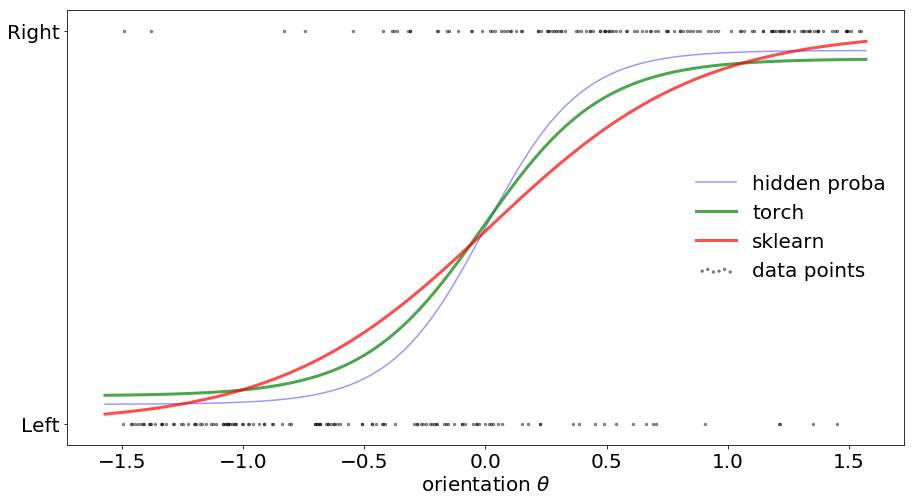There is a clear discrepency between the classical logistic function obtained by logistic regression (here, the implementation by sklearn) and that obtained by our more detailed model. In particular, the slope is more sharp, a feature which may be important for cognitive processes. But what is the origin of this discrepancy? Is it the numerical optimization method? Is it the generative model?

This are the question we try to resolve here. First, let's retrieve a measure for how well the extracted psychometric curve represent the data: the losses.

In :
theta, p, y = get_data(N=N_test, seed=seed + N_test)

In :
logistic_model, loss = fit_data(theta, y, verbose=False)
print(f"Training loss = {loss:.3f}")

Training loss = 0.330

In :
logistic_model_sk, loss_sk = fit_data_sklearn(theta, y, verbose=False)
print(f"Training sklearn loss = {loss_sk:.3f}")

Training sklearn loss = 0.350


The units for these losses are bits. Indeed, they represent some value of information about the binary data "$d$" and the continuous psychometric curve "$p$". This information is equal to $\log_2(p)$ if $d=1$ and $\log_2(1-p)$ if $d=0$. Putting things together, we obtain the Binary Cross Entropy and if we index datapoints by $k$:

$$\mathcal{L} = \sum_k d_k \cdot \log_2(p_k) + (1-d_k) \cdot \log_2(1-p_k)$$

You can see that this measure is a form of Cross_entropy, adapted to binary datapoints.

The losses which were computed above are those obtained during training. Relying on this value may be a dangerous strategy as the model may be overfitting the data. We should therefore measure how the model would generalize with novel data.

While it hard to do with real (experimental) data which are often scarse, here we synthesized the data and we can thus compute a testing loss by drawing again a set of new data and computing the loss on that data:

In :
def loss_torch(logistic_model, theta, p, y):
labels = torch.Tensor(y[:, None])
Theta = torch.Tensor(theta[:, None])
P = torch.Tensor(p[:, None])

outputs = logistic_model(Theta)
return criterion(outputs, labels).item() / len(theta)

print(f"Testing loss = {loss_torch(logistic_model, theta, p, y):.3f}")

Testing loss = 0.330

In :
def loss_sklearn(logistic_model, theta, p, y):
outputs = logistic_model.predict_proba(theta[:, None])[:, 1]
outputs_, labels = torch.Tensor(outputs[:, None]), torch.Tensor(y[:, None])
return criterion(outputs_, labels).item() / len(theta)

print(f"Testing sklearn loss = {loss_sklearn(logistic_model_sk, theta, p, y):.3f}")

Testing sklearn loss = 0.350


Finally, as we synthesized the data, we can compute the loss on that data with the "true" psychometric curve, giving to us the baseline number one can achieve:

In :
def loss_true(theta, p, y):
labels = torch.Tensor(y[:, None])
P = torch.Tensor(p[:, None])
return criterion(P, labels).item() / len(theta)

print(f"Testing true loss = {loss_true(theta, p, y):.3f}")

Testing true loss = 0.331


We are now equipped to make quantitative comparisons. Let's first explore the parameters of the methods.

### quantitative comparison of methods : varrying methods' parameters¶

Let's study the influence of each method's meta-parameter, such as the number of iterations. As I learn to use python decorators, I will use plenty of them:

In :
import functools
import time

def timer(func):
"""Print the runtime of the decorated function"""
@functools.wraps(func)
def wrapper_timer(*args, **kwargs):
start_time = time.perf_counter()    # 1
results = func(*args, **kwargs)
end_time = time.perf_counter()      # 2
run_time = end_time - start_time    # 3
print(f"Finished {func.__name__!r} in {run_time:.4f} secs")
return results
return wrapper_timer


#### influence of learning rate¶

In :
default_dict = dict(learning_rate=learning_rate,
batch_size=batch_size,  # gamma=gamma,
num_epochs=num_epochs,
betas=betas,
N=N, p0=p0, theta0=theta0, wt=wt, theta_std=theta_std, seed=seed)

In :
@timer
def explore_param(default_dict, variable, var_range, do_fit=True, do_SKL=True):
results = np.zeros((4, len(var_range), N_cv))
timings = []

for i_var, var_ in enumerate(var_range):
kwarg = default_dict.copy()
kwarg[variable] = var_
kwarg['verbose'] = False

for i_CV in range(N_cv):

kwarg.update(seed=seed + i_CV)
theta, p, y = get_data(**kwarg)

tic = time.time()
if do_fit: logistic_model, loss = fit_data(theta, y, **kwarg)
if do_SKL: logistic_model_sk, loss_SKL = fit_data_sklearn(theta, y, **kwarg)
toc = time.time()

if N_test > 0:
kwarg.update(N=N_test, seed=seed + i_CV + N_test)
theta, p, y = get_data(**kwarg)
if do_fit: loss = loss_torch(logistic_model, theta, p, y)
if do_SKL: loss_SKL = loss_sklearn(logistic_model_sk, theta, p, y)

results[0, i_var, i_CV] = loss_true(theta, p, y)
if do_fit: results[1, i_var, i_CV] = loss
if do_SKL: results[2, i_var, i_CV] = loss_SKL
results[3, i_var, i_CV] = toc-tic

print(f"{variable}: {var_:.5f}, Loss: {np.mean(results[1, i_var, :]):.5f}, loss_P: {np.mean(results[0, i_var, :]):.5f}")

return results


In :
learning_rates = learning_rate * np.logspace(-2, 1, N_scan, base=10)
results = explore_param(default_dict, variable='learning_rate', var_range=learning_rates, do_SKL=False)

learning_rate: 0.00005, Loss: 0.34094, loss_P: 0.33333
learning_rate: 0.00012, Loss: 0.33752, loss_P: 0.33333
learning_rate: 0.00028, Loss: 0.33505, loss_P: 0.33333
learning_rate: 0.00067, Loss: 0.33428, loss_P: 0.33333
learning_rate: 0.00158, Loss: 0.33466, loss_P: 0.33333
learning_rate: 0.00375, Loss: 0.33497, loss_P: 0.33333
learning_rate: 0.00889, Loss: 0.33502, loss_P: 0.33333
learning_rate: 0.02108, Loss: 0.33512, loss_P: 0.33333
learning_rate: 0.05000, Loss: 0.33510, loss_P: 0.33333
Finished 'explore_param' in 1050.0953 secs

In :
def plot_explore_param(variable, var_range, results):
opts_scat = dict(marker=".", lw=0, alpha=3 / N_cv, ms=20)
opts_line = dict(lw=1, alpha=1)

fig, ax = plt.subplots(figsize=(15, 8))
if results[1, ...].sum()>0: ax.plot(var_range, results[1, :, :]/results[0, :, :], **opts_scat, color="green")
ax.plot(var_range, results[0, :, :]/results[0, :, :], **opts_scat, color="blue")
if results[2, ...].sum()>0: ax.plot(var_range, results[2, :, :]/results[0, :, :], **opts_scat, color="red")

if results[1, ...].sum()>0: ax.plot(var_range, np.mean(results[1, :, :]/results[0, :, :], axis=-1), **opts_line, color="green", label="loss")
ax.plot(var_range, np.mean(results[0, :, :]/results[0, :, :], axis=-1), **opts_line, color="blue", label="true")
if results[2, ...].sum()>0: ax.plot(var_range, np.mean(results[2, :, :]/results[0, :, :], axis=-1), **opts_line, color="red", label="loss_SKL")

# ax.set_xlim(np.min(learning_rates_), np.max(learning_rates_))

ax.set_xlabel(variable)
ax.set_ylabel("Loss")
ax.set_xscale("log")
ax.legend(loc="best")
return fig, ax

fig, ax = plot_explore_param(variable='learning_rate', var_range=learning_rates, results=results)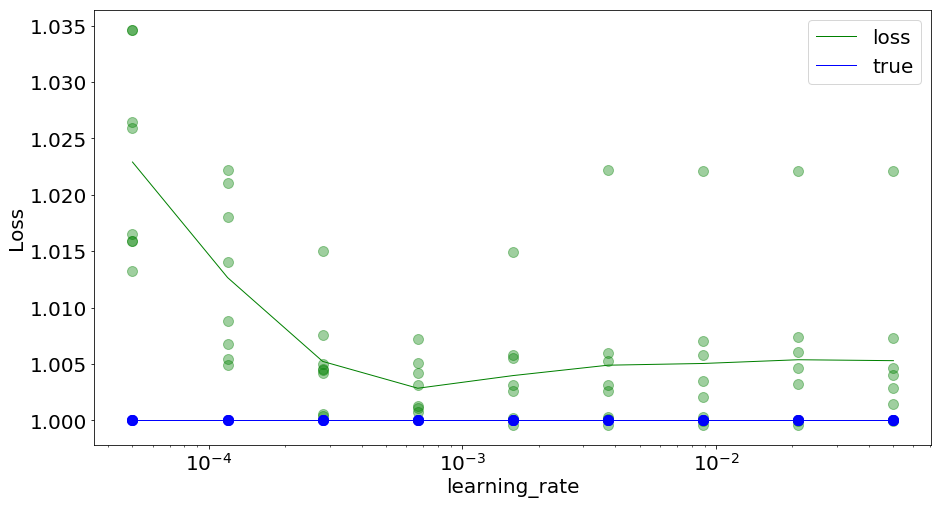We are at a sweet spot with our learning rate, still it is valid on a wide range.

#### influence du nombre d'epochs¶

In :
num_epochss = num_epochs * np.logspace(-2, 1, N_scan, base=10)
num_epochss = [int(num_epochs_) for num_epochs_ in num_epochss]

results = explore_param(default_dict, variable='num_epochs', var_range=num_epochss, do_SKL=False)

num_epochs: 5.00000, Loss: 0.34121, loss_P: 0.33333
num_epochs: 12.00000, Loss: 0.33748, loss_P: 0.33333
num_epochs: 28.00000, Loss: 0.33510, loss_P: 0.33333
num_epochs: 68.00000, Loss: 0.33435, loss_P: 0.33333
num_epochs: 162.00000, Loss: 0.33477, loss_P: 0.33333
num_epochs: 384.00000, Loss: 0.33500, loss_P: 0.33333
num_epochs: 912.00000, Loss: 0.33500, loss_P: 0.33333
num_epochs: 2163.00000, Loss: 0.33495, loss_P: 0.33333
num_epochs: 5130.00000, Loss: 0.33504, loss_P: 0.33333
Finished 'explore_param' in 2290.9014 secs

In :
fig, ax = plot_explore_param(variable='num_epochs', var_range=num_epochss, results=results)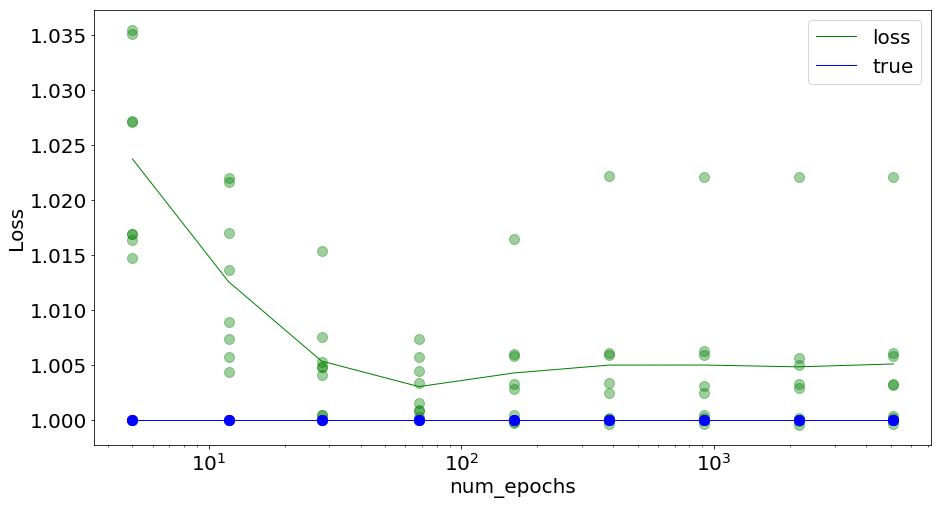Similarly, we are at a sweet spot with our number of epochs, and this is true for both methods.

#### influence of minibatch size¶

In :
import time

In :
batch_sizes = N * np.logspace(-3, 0, N_scan, base=2)
batch_sizes = [int(batch_size_) for batch_size_ in batch_sizes]

results = explore_param(default_dict, variable='batch_size', var_range=batch_sizes, do_SKL=False)

batch_size: 32.00000, Loss: 0.33508, loss_P: 0.33333
batch_size: 41.00000, Loss: 0.33488, loss_P: 0.33333
batch_size: 53.00000, Loss: 0.33498, loss_P: 0.33333
batch_size: 69.00000, Loss: 0.33494, loss_P: 0.33333
batch_size: 90.00000, Loss: 0.33501, loss_P: 0.33333
batch_size: 117.00000, Loss: 0.33489, loss_P: 0.33333
batch_size: 152.00000, Loss: 0.33496, loss_P: 0.33333
batch_size: 197.00000, Loss: 0.33497, loss_P: 0.33333
batch_size: 256.00000, Loss: 0.33498, loss_P: 0.33333
Finished 'explore_param' in 1650.6039 secs

In :
fig, ax = plot_explore_param(variable='batch_size', var_range=batch_sizes, results=results)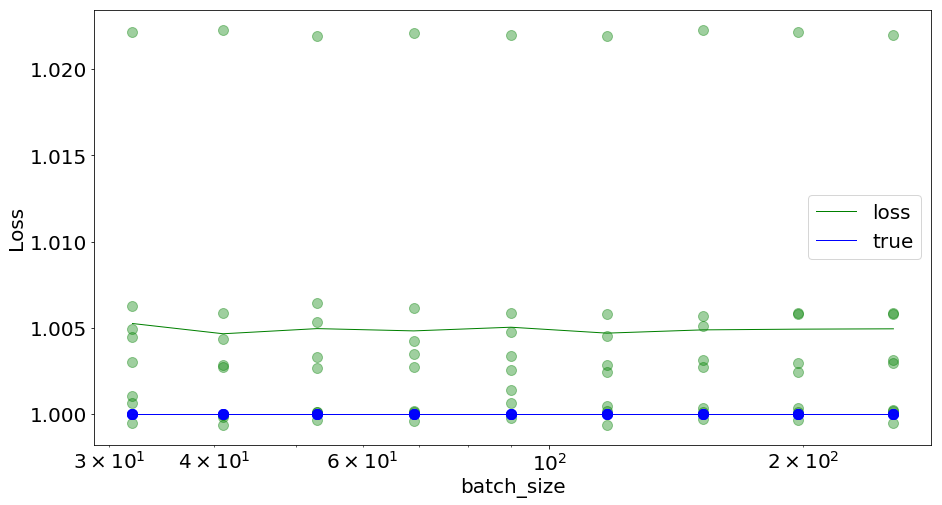As expected, batch_size as minor effects on the cost, but it can on the CPU time:

In :
fig, ax = plt.subplots(figsize=(15, 8))
opts_scat = dict(marker=".", lw=0, alpha=.9, ms=20)
ax.plot(batch_sizes, np.mean(results[3, :, :], axis=-1), **opts_scat)
ax.set_xlabel("batch_size")
ax.set_ylabel("CPU time (s)")
ax.set_ylim(0)
ax.set_xscale("log");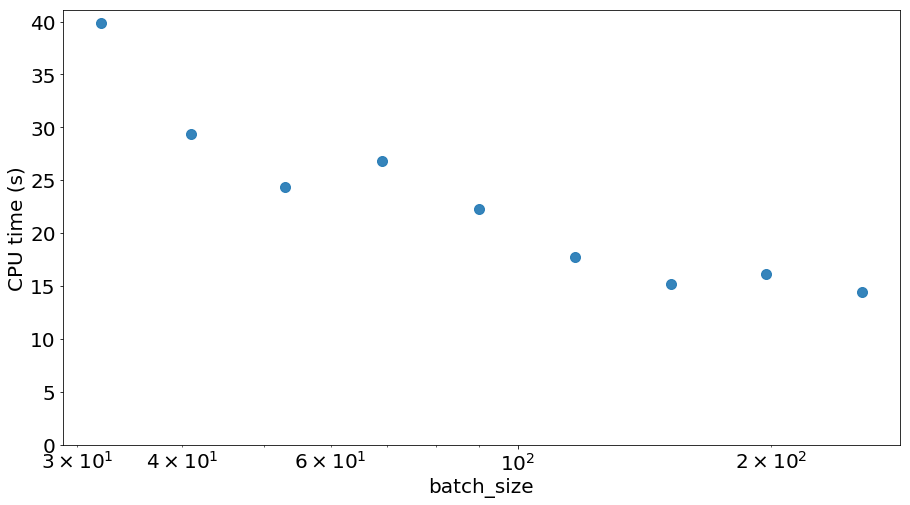#### influence of beta1¶

In :
beta1s = 1.0 - np.logspace(-3, -2, N_scan, base=10, endpoint=True)
results = explore_param(default_dict, variable='beta1', var_range=beta1s, do_SKL=False)

beta1: 0.99900, Loss: 0.33501, loss_P: 0.33333
beta1: 0.99867, Loss: 0.33496, loss_P: 0.33333
beta1: 0.99822, Loss: 0.33495, loss_P: 0.33333
beta1: 0.99763, Loss: 0.33495, loss_P: 0.33333
beta1: 0.99684, Loss: 0.33500, loss_P: 0.33333
beta1: 0.99578, Loss: 0.33498, loss_P: 0.33333
beta1: 0.99438, Loss: 0.33498, loss_P: 0.33333
beta1: 0.99250, Loss: 0.33501, loss_P: 0.33333
beta1: 0.99000, Loss: 0.33499, loss_P: 0.33333
Finished 'explore_param' in 1129.4505 secs

In :
fig, ax = plot_explore_param(variable='beta1', var_range=beta1s, results=results)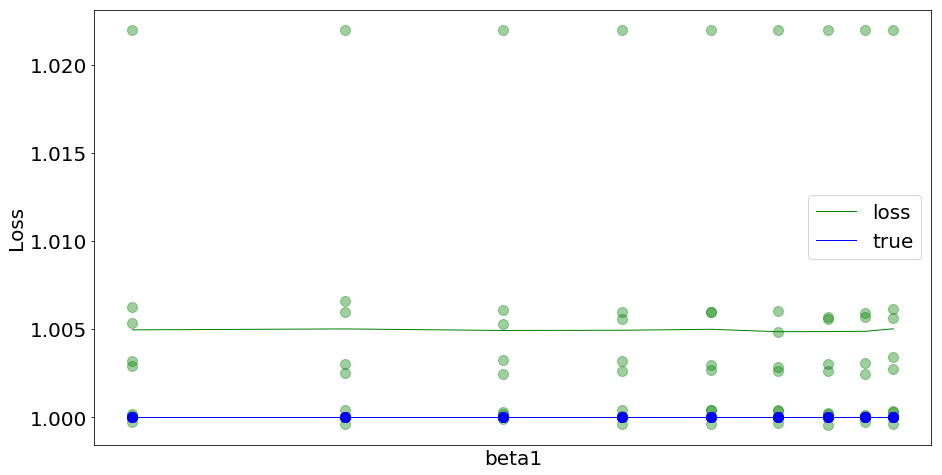The influence of this parameter is limited, such that using Adam is perhaps overkill. A simple SGD should be tested. Similarly, for beta2:

#### influence of beta2¶

In :
beta2s = 1.0 - np.logspace(-7, -2, N_scan, base=10, endpoint=True)
results = explore_param(default_dict, variable='beta2', var_range=beta2s, do_SKL=False)

beta2: 1.00000, Loss: 0.33498, loss_P: 0.33333
beta2: 1.00000, Loss: 0.33495, loss_P: 0.33333
beta2: 1.00000, Loss: 0.33496, loss_P: 0.33333
beta2: 0.99999, Loss: 0.33495, loss_P: 0.33333
beta2: 0.99997, Loss: 0.33498, loss_P: 0.33333
beta2: 0.99987, Loss: 0.33498, loss_P: 0.33333
beta2: 0.99944, Loss: 0.33499, loss_P: 0.33333
beta2: 0.99763, Loss: 0.33497, loss_P: 0.33333
beta2: 0.99000, Loss: 0.33499, loss_P: 0.33333
Finished 'explore_param' in 1153.4729 secs

In :
fig, ax = plot_explore_param(variable='beta2', var_range=beta2s, results=results)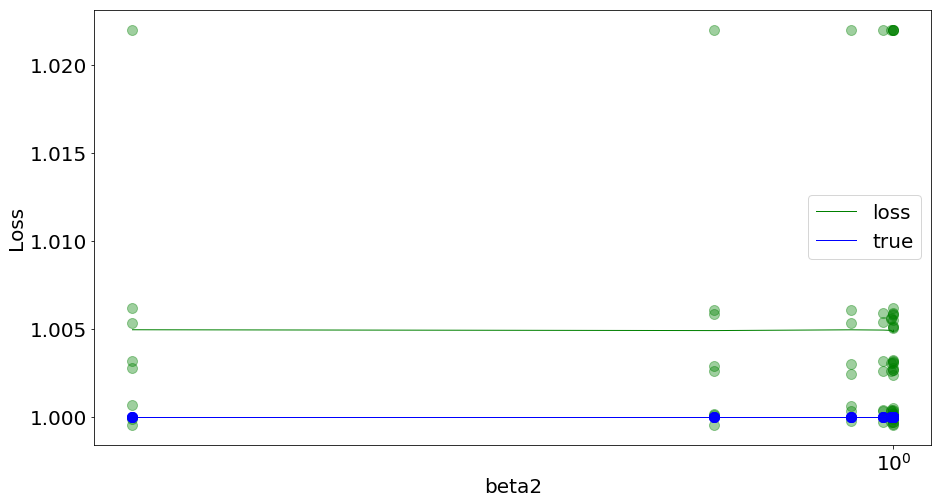#### influence of C¶

In :
Cs = C * np.logspace(-2, 2, N_scan, base=4)
results = explore_param(default_dict, variable='C', var_range=Cs, do_fit=False)

C: 0.18750, Loss: 0.00000, loss_P: 0.33333
C: 0.37500, Loss: 0.00000, loss_P: 0.33333
C: 0.75000, Loss: 0.00000, loss_P: 0.33333
C: 1.50000, Loss: 0.00000, loss_P: 0.33333
C: 3.00000, Loss: 0.00000, loss_P: 0.33333
C: 6.00000, Loss: 0.00000, loss_P: 0.33333
C: 12.00000, Loss: 0.00000, loss_P: 0.33333
C: 24.00000, Loss: 0.00000, loss_P: 0.33333
C: 48.00000, Loss: 0.00000, loss_P: 0.33333
Finished 'explore_param' in 0.5097 secs

In :
fig, ax = plot_explore_param(variable='C', var_range=Cs, results=results)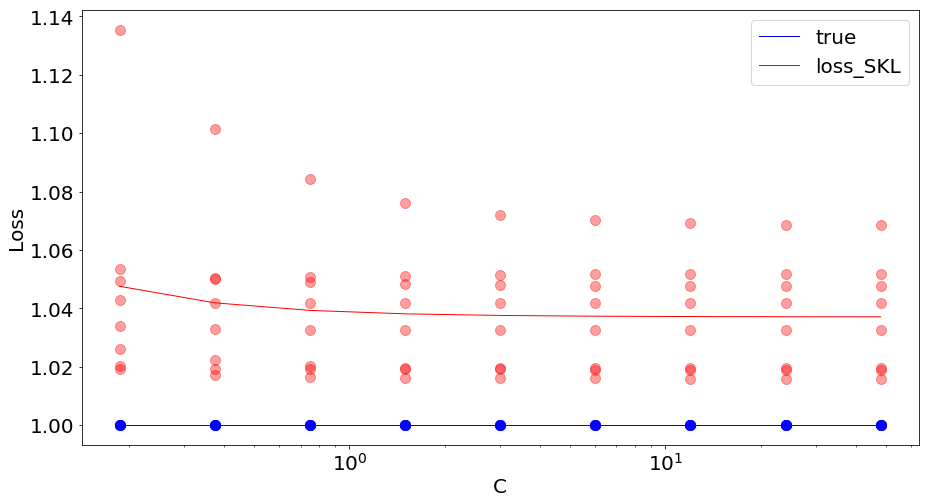#### influence of tol¶

In :
tols = tol * np.logspace(-2, 2, N_scan, base=10)
results = explore_param(default_dict, variable='tol', var_range=tols, do_fit=False)

tol: 0.00000, Loss: 0.00000, loss_P: 0.33333
tol: 0.00000, Loss: 0.00000, loss_P: 0.33333
tol: 0.00000, Loss: 0.00000, loss_P: 0.33333
tol: 0.00000, Loss: 0.00000, loss_P: 0.33333
tol: 0.00001, Loss: 0.00000, loss_P: 0.33333
tol: 0.00003, Loss: 0.00000, loss_P: 0.33333
tol: 0.00010, Loss: 0.00000, loss_P: 0.33333
tol: 0.00032, Loss: 0.00000, loss_P: 0.33333
tol: 0.00100, Loss: 0.00000, loss_P: 0.33333
Finished 'explore_param' in 0.3474 secs

In :
fig, ax = plot_explore_param(variable='tol', var_range=tols, results=results)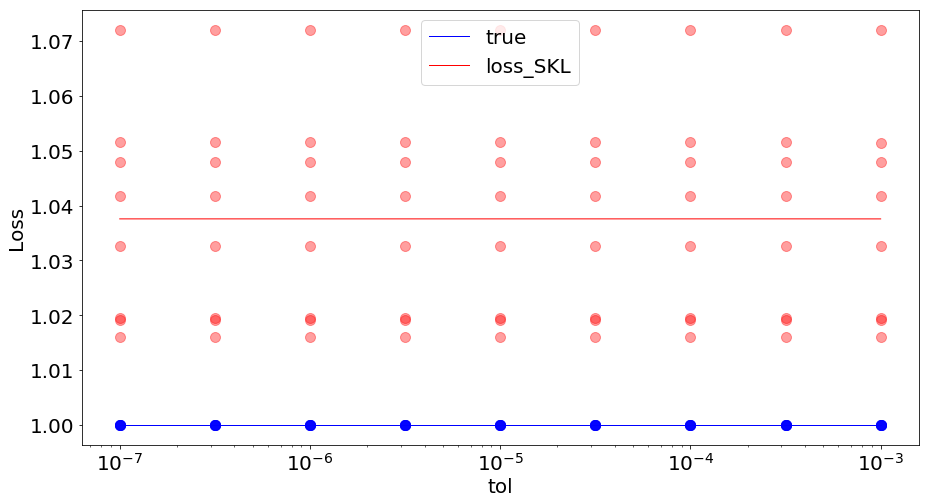### quantitative comparison of methods : varrying experimental parameters¶

Now that we now more about methodological parameters, let's study more crucial parameters like that of the experiment.

#### influence of number of trials¶

The number of trials is crucial as if defines the number of our datapoints, and also the length of the experiment. This is important as we want to make this number as low as possible. Indeed, observers doing the experiment, for example a master student in front of the computer screen, may experience fatigue which would prevent accurate recordings.

In :
Ns = np.logspace(1.5, 3, N_scan, base=10, endpoint=True)
Ns = [int(Ns_) for Ns_ in Ns]

results = explore_param(default_dict, variable='N', var_range=Ns)

N: 31.00000, Loss: 0.34505, loss_P: 0.33333
N: 48.00000, Loss: 0.33679, loss_P: 0.33333
N: 74.00000, Loss: 0.33690, loss_P: 0.33333
N: 115.00000, Loss: 0.33860, loss_P: 0.33333
N: 177.00000, Loss: 0.33495, loss_P: 0.33333
N: 273.00000, Loss: 0.33436, loss_P: 0.33333
N: 421.00000, Loss: 0.33410, loss_P: 0.33333
N: 649.00000, Loss: 0.33419, loss_P: 0.33333
N: 1000.00000, Loss: 0.33411, loss_P: 0.33333
Finished 'explore_param' in 1402.8436 secs

In :
fig, ax = plot_explore_param(variable='N', var_range=Ns, results=results)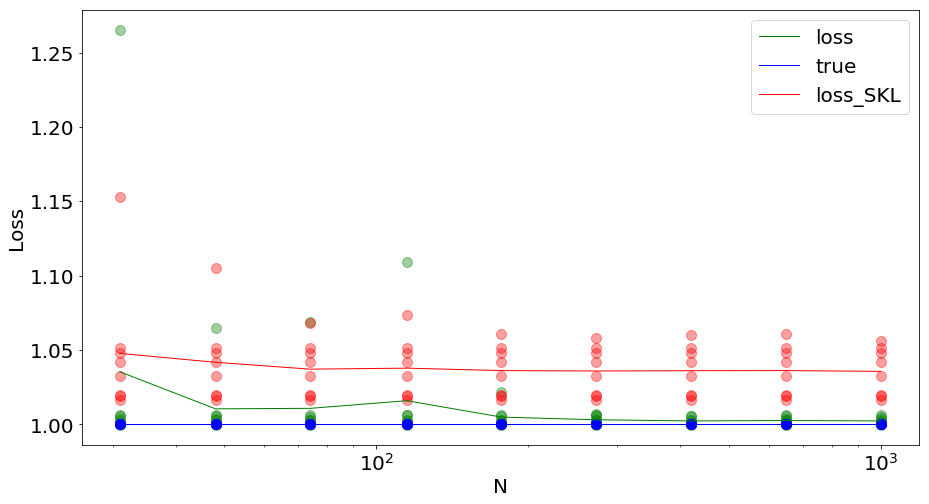As a consequence, $20$ trials is not enough and $100$ is OK. This depends on our expectations on the the retrieved data.

#### influence of theta_std¶

The convergence of the fitting procedure may also depend on the parametrers of the data which were set to:

In :
print(
f"p0 = {p0:.3f}, theta0 = {theta0:.3f}, wt = {wt:.3f}, theta_std = {theta_std:.3f}"
)

p0 = 0.100, theta0 = 0.000, wt = 0.196, theta_std = 1.571


One of this is theta_std and is describing the "width" of tested orientation values:

In :
theta_stds = theta_std * np.logspace(-1, 1, N_scan, base=2, endpoint=True)

results = explore_param(default_dict, variable='theta_std', var_range=theta_stds)

theta_std: 0.78540, Loss: 0.45484, loss_P: 0.45436
theta_std: 0.93400, Loss: 0.42213, loss_P: 0.42138
theta_std: 1.11072, Loss: 0.38933, loss_P: 0.38805
theta_std: 1.32088, Loss: 0.36055, loss_P: 0.35931
theta_std: 1.57080, Loss: 0.33497, loss_P: 0.33333
theta_std: 1.86800, Loss: 0.31435, loss_P: 0.31297
theta_std: 2.22144, Loss: 0.29658, loss_P: 0.29535
theta_std: 2.64175, Loss: 0.28170, loss_P: 0.28050
theta_std: 3.14159, Loss: 0.27050, loss_P: 0.26943
Finished 'explore_param' in 1175.3516 secs

In :
fig, ax = plot_explore_param(variable='theta_std', var_range=theta_stds, results=results)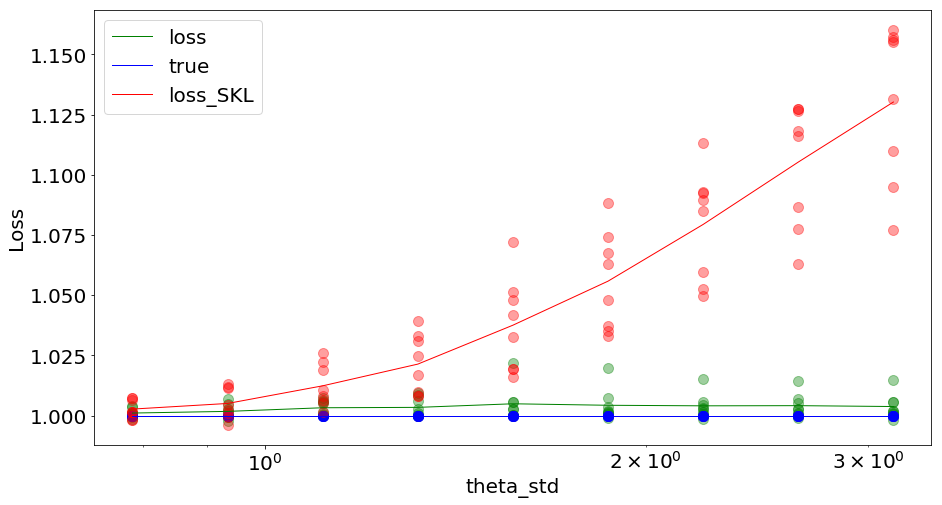The value of theta_std as a clear influence on the loss in particular for classical logistic regression (sklearn) which will have a problem with datapoints caused by the lapse rate p0.

#### influence of p0¶

In :
p0s = np.logspace(-3, -0.7, N_scan, base=10, endpoint=True)

results = explore_param(default_dict, variable='p0', var_range=p0s)

p0: 0.00100, Loss: 0.20977, loss_P: 0.20832
p0: 0.00194, Loss: 0.21225, loss_P: 0.21004
p0: 0.00376, Loss: 0.21517, loss_P: 0.21328
p0: 0.00729, Loss: 0.22231, loss_P: 0.22059
p0: 0.01413, Loss: 0.23155, loss_P: 0.22954
p0: 0.02738, Loss: 0.25081, loss_P: 0.24829
p0: 0.05309, Loss: 0.28281, loss_P: 0.28041
p0: 0.10292, Loss: 0.33746, loss_P: 0.33586
p0: 0.19953, Loss: 0.42551, loss_P: 0.42388
Finished 'explore_param' in 897.9082 secs

In :
fig, ax = plot_explore_param(variable='p0', var_range=p0s, results=results)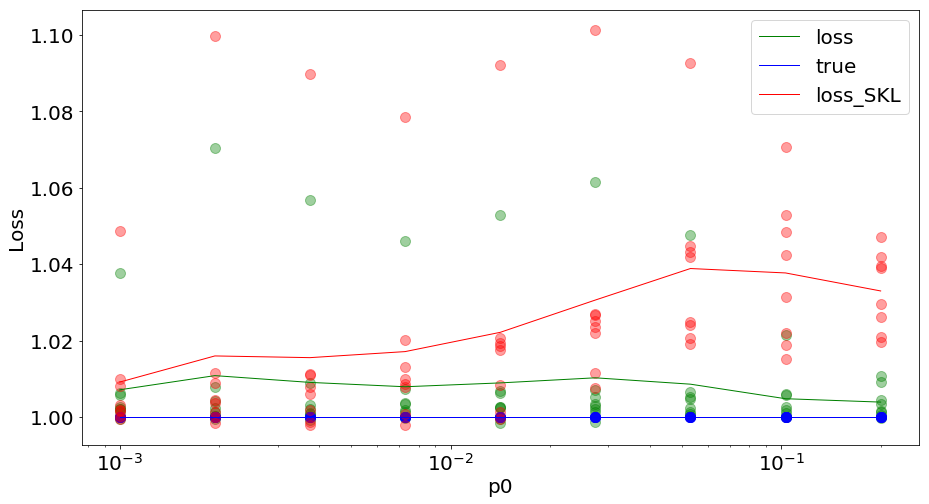As expected, the lapse rate p0as an influence on the cost: when low ($p0<0.01$), cost are similar. When higher, the two methods diverge and our method is obviously better.

After this quantitative comparison of the methods, let's now study how the methods compare when retrieving the parameters.

### comparing the predicted values¶

In this section, we will change one parameter after antoher, while keeping the others fixed and check the retrieved value obtained by both methods.

In :
print(
f"p0 = {p0:.3f}, theta0 = {theta0:.3f}, wt = {wt:.3f}, theta_std = {theta_std:.3f}"
)

p0 = 0.100, theta0 = 0.000, wt = 0.196, theta_std = 1.571


#### changing p0¶

Let's start by changing the lapse rate p0:

In :
N_scan = 20
p0s = np.logspace(-3, -0.7, N_scan, base=10, endpoint=True)

p0s_, wts_, theta0s_, p0_tos, theta0_tos, theta0_sks, wt_tos, wt_sks = (
[],
[],
[],
[],
[],
[],
[],
[],
)

for p0_ in p0s:
for i_CV in range(N_cv):
theta, p, y = get_data(p0=p0_, seed=seed + i_CV)

logistic_model, loss = fit_data(theta, y, verbose=False)
logistic_model_sk, loss_SKL = fit_data_sklearn(theta, y, verbose=False)

theta0_to, wt_to, p0_to = get_params(logistic_model, verbose=False)
theta0_sk, wt_sk = get_params_sk(logistic_model_sk, verbose=False)

p0s_.append(p0_)
theta0s_.append(theta0)
wts_.append(wt)
p0_tos.append(p0_to)
theta0_tos.append(theta0_to)
theta0_sks.append(theta0_sk)
wt_tos.append(wt_to)
wt_sks.append(wt_sk)

In :
fig, axs = plt.subplots(1, 3, figsize=(15, 8))

axs.scatter(p0s_, p0_tos, label="torch")
axs.plot([min(p0s_), max(p0s_)], [min(p0_tos), max(p0_tos)], "--")
axs.set(xlabel="p0 (true)", ylabel="p0 (predicted)")
axs.legend(loc="upper left")

axs.scatter(p0s_, theta0_tos, label="torch")
axs.scatter(p0s_, theta0_sks, label="sklearn")
axs.plot([min(p0s_), max(p0s_)], [theta0, theta0], "--")
axs.set(xlabel="p0", ylabel="theta0 (predicted)")
axs.legend(loc="upper left")

axs.scatter(p0s_, wt_tos, label="torch")
axs.scatter(p0s_, wt_sks, label="sklearn")
axs.plot([min(p0s_), max(p0s_)], [wt, wt], "--")
axs.set(xlabel="p0", ylabel="slope (predicted)")
axs.legend(loc="upper left")
plt.tight_layout();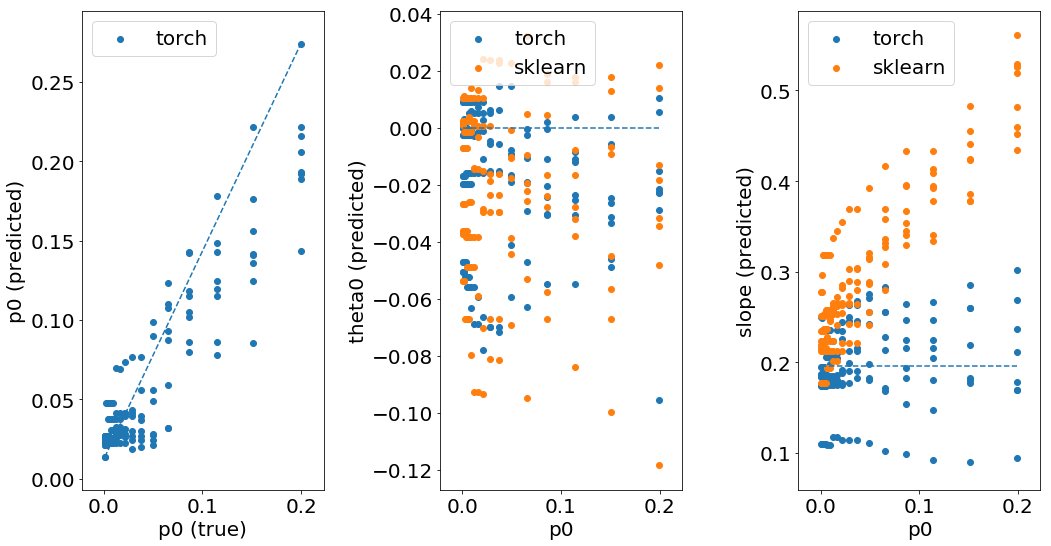Our method is able to fairly accurately retrieve the value of the lapse rate. The errors obtained in the fitting of the other parameters are comparable forr theta0but are high on the slope. The slope is clearly overestimated depending on the lapse rate.

#### changing theta0¶

In :
N_scan = 20
theta0s = .61803 * theta_std * np.linspace(-1, 1, N_scan, endpoint=True)

p0s_, wts_, theta0s_, p0_tos, theta0_tos, theta0_sks, wt_tos, wt_sks = (
[],
[],
[],
[],
[],
[],
[],
[],
)

for theta0_ in theta0s:
for i_CV in range(N_cv):
theta, p, y = get_data(theta0=theta0_, seed=seed + i_CV)

logistic_model, loss = fit_data(theta, y, verbose=False)
logistic_model_sk, loss_SKL = fit_data_sklearn(theta, y, verbose=False)

theta0_to, wt_to, p0_to = get_params(logistic_model, verbose=False)
theta0_sk, wt_sk = get_params_sk(logistic_model_sk, verbose=False)

p0s_.append(p0)
theta0s_.append(theta0_)
wts_.append(wt)
p0_tos.append(p0_to)
theta0_tos.append(theta0_to)
theta0_sks.append(theta0_sk)
wt_tos.append(wt_to)
wt_sks.append(wt_sk)

In :
fig, axs = plt.subplots(1, 3, figsize=(15, 8))

axs.scatter(theta0s_, p0_tos, label="torch")
axs.plot([min(theta0s_), max(theta0s_)], [p0, p0], "--", label="true")
axs.set(xlabel="theta0 (true)", ylabel="p0 (predicted)", ylim=(.0, .2))
axs.legend(loc="upper left")

axs.scatter(theta0s_, theta0_tos, label="torch")
axs.scatter(theta0s_, theta0_sks, label="sklearn")
axs.plot([min(theta0s_), max(theta0s_)], [min(theta0s_), max(theta0s_)], "--", label="true")
axs.set(xlabel="theta0 (true)", ylabel="theta0 (predicted)")
axs.legend(loc="upper left")

axs.scatter(theta0s_, wt_tos, label="torch")
axs.scatter(theta0s_, wt_sks, label="sklearn")
axs.plot([min(theta0s_), max(theta0s_)], [wt, wt], "--", label="true")
axs.set(xlabel="theta0 (true)", ylabel="slope (predicted)")
axs.legend(loc="upper left")
plt.tight_layout();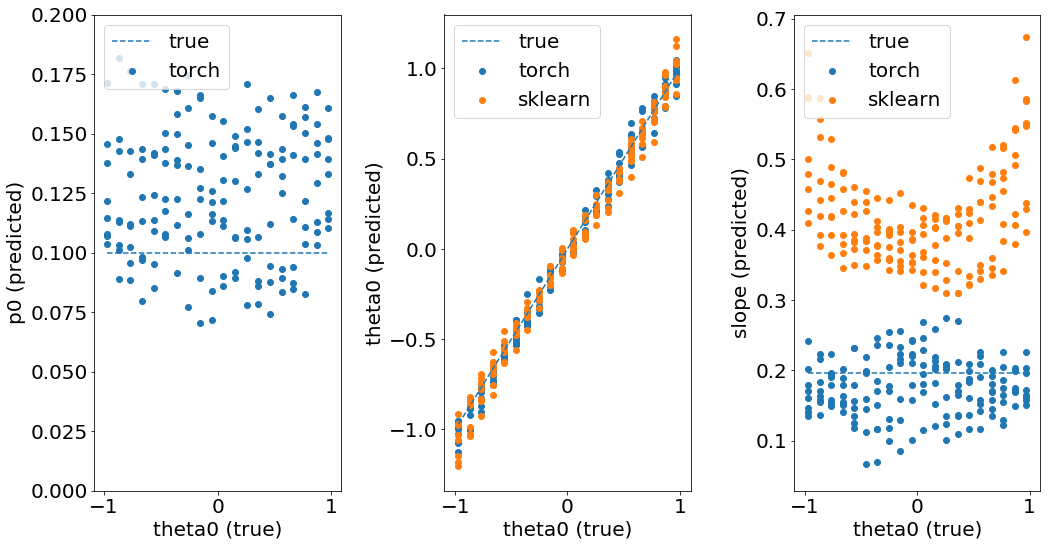#### changing wt¶

In :
N_scan = 20
wts = wt * np.logspace(-1, .5, N_scan, base=4, endpoint=True)

p0s_, wts_, theta0s_, p0_tos, theta0_tos, theta0_sks, wt_tos, wt_sks = (
[],
[],
[],
[],
[],
[],
[],
[],
)

for wt_ in wts:
for i_CV in range(N_cv):
theta, p, y = get_data(wt=wt_, seed=seed + i_CV)

logistic_model, loss = fit_data(theta, y, verbose=False)
logistic_model_sk, loss_SKL = fit_data_sklearn(theta, y, verbose=False)

theta0_to, wt_to, p0_to = get_params(logistic_model, verbose=False)
theta0_sk, wt_sk = get_params_sk(logistic_model_sk, verbose=False)

p0s_.append(p0)
theta0s_.append(theta0)
wts_.append(wt_)
p0_tos.append(p0_to)
theta0_tos.append(theta0_to)
theta0_sks.append(theta0_sk)
wt_tos.append(wt_to)
wt_sks.append(wt_sk)

In :
fig, axs = plt.subplots(1, 3, figsize=(15, 8))

axs.scatter(wts_, p0_tos, label="torch")
axs.plot([min(wts_), max(wts_)], [p0, p0], "--", label="true")
axs.set(xlabel="slope (true)", ylabel="p0 (predicted)", ylim=(.0, .3))
axs.legend(loc="upper left")

axs.scatter(wts_, theta0_tos, label="torch")
axs.scatter(wts_, theta0_sks, label="sklearn")
axs.plot([min(wts_), max(wts_)], [theta0, theta0], "--", label="true")
axs.set(xlabel="slope (true)", ylabel="theta0 (predicted)", ylim=(-.2, .2))
axs.legend(loc="upper left")

axs.scatter(wts_, wt_tos, label="torch")
axs.scatter(wts_, wt_sks, label="sklearn")
axs.plot([min(wts_), max(wts_)], [min(wts_), max(wts_)], "--", label="true")
axs.set(xlabel="slope (true)", ylabel="slope (predicted)")
axs.legend(loc="upper left")
plt.tight_layout();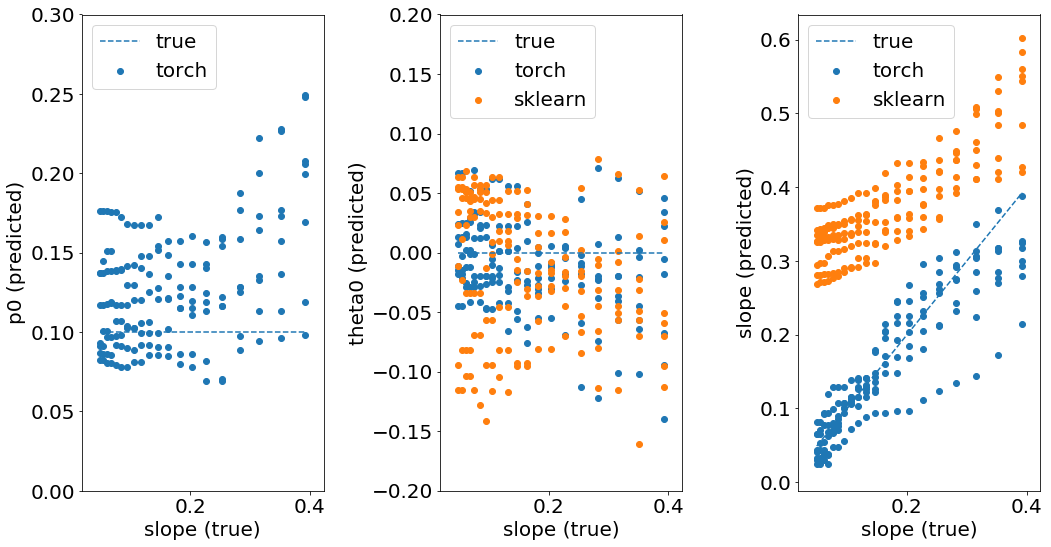Our method is able to fairly accurately retrieve the value of the lapse rate, but the precision decreases with the lapse rate. The errors obtained in the fitting of the other parameters are comparable forr theta0 but are high on the slope. The slope is clearly overestimated in the case of the classical logistic regression.

#### changing wt with a null lapse rate (p0=0)¶

Let's decompose the effect of using torch to using sklearn

In :
N_scan = 20
wts = wt * np.logspace(-1, .5, N_scan, base=4, endpoint=True)

p0s_, wts_, theta0s_, p0_tos, theta0_tos, theta0_sks, wt_tos, wt_sks = (
[],
[],
[],
[],
[],
[],
[],
[],
)

for wt_ in wts:
for i_CV in range(N_cv):
theta, p, y = get_data(wt=wt_, p0=0, seed=seed + i_CV)

logistic_model, loss = fit_data(theta, y, verbose=False)
logistic_model_sk, loss_SKL = fit_data_sklearn(theta, y, verbose=False)

theta0_to, wt_to, p0_to = get_params(logistic_model, verbose=False)
theta0_sk, wt_sk = get_params_sk(logistic_model_sk, verbose=False)

p0s_.append(p0)
theta0s_.append(theta0)
wts_.append(wt_)
p0_tos.append(p0_to)
theta0_tos.append(theta0_to)
theta0_sks.append(theta0_sk)
wt_tos.append(wt_to)
wt_sks.append(wt_sk)

In :
fig, axs = plt.subplots(1, 3, figsize=(15, 8))

axs.scatter(wts_, p0_tos, label="torch")
axs.plot([min(wts_), max(wts_)], [0, 0], "--", label="true")
axs.set(xlabel="slope (true)", ylabel="p0 (predicted)")
axs.legend(loc="upper left")

axs.scatter(wts_, theta0_tos, label="torch")
axs.scatter(wts_, theta0_sks, label="sklearn")
axs.plot([min(wts_), max(wts_)], [theta0, theta0], "--", label="true")
axs.set(xlabel="slope (true)", ylabel="theta0 (predicted)", ylim=(-.2, .2))
axs.legend(loc="upper left")

axs.scatter(wts_, wt_tos, label="torch")
axs.scatter(wts_, wt_sks, label="sklearn")
axs.plot([min(wts_), max(wts_)], [min(wts_), max(wts_)], "--", label="true")
axs.set(xlabel="slope (true)", ylabel="slope (predicted)")
axs.legend(loc="upper left")
plt.tight_layout();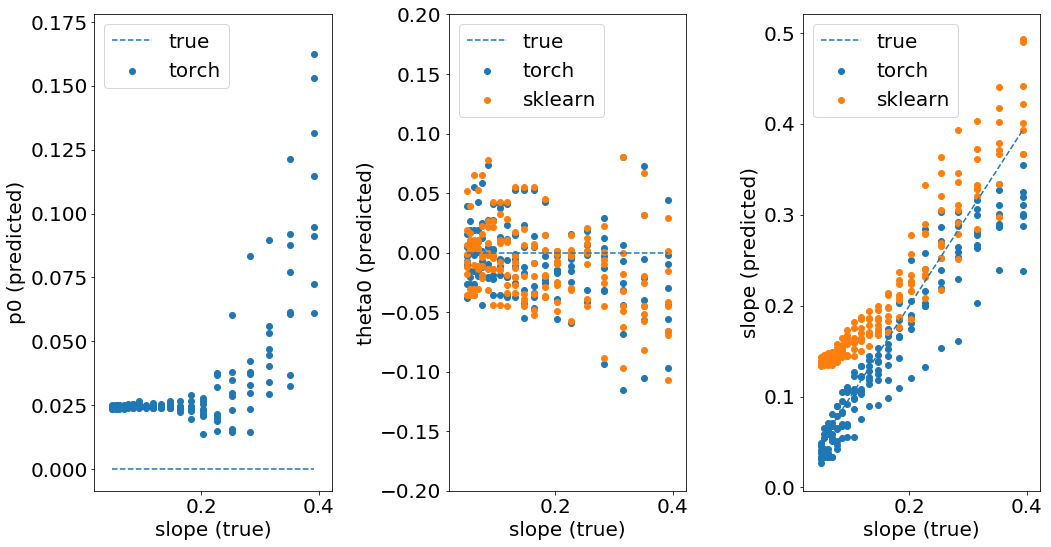This again shows that the slope is better matched with the method presented in this notebook.

In :
%load_ext watermark
%watermark -i -h -m -v -p numpy,torch,sklearn,matplotlib  -r -g -b

2020-04-15T12:44:35+02:00

CPython 3.7.7
IPython 7.13.0

numpy 1.18.2
torch 1.4.0
sklearn 0.22.2.post1
matplotlib 3.2.1

compiler   : Clang 11.0.0 (clang-1100.0.33.17)
system     : Darwin
release    : 19.4.0
machine    : x86_64
processor  : i386
CPU cores  : 4
interpreter: 64bit
host name  : ekla
Git hash   : e67413e87371bea2e333f61d02b91e0c647ac9a2